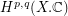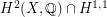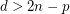# The Hodge Conjecture

 Importance: Outstanding ✭✭✭✭
 Author(s): Hodge, W. V. D.
 Subject: Geometry » Algebraic Geometry
 Keywords: Hodge Theory Millenium Problems
 Prize: \$1,000,000 from the Clay Mathematics Institute
 Posted by: Charles on: July 13th, 2008
Conjecture   Letbe a complex projective variety. Then every Hodge class is a rational linear combination of the cohomology classes of complex subvarieties of.

A complex projective variety is the set of zeros of a finite collection of homogeneous polynomials on projective space, and we are concerned with the singular cohomology ring. There is a well known Hodge Decomposition of the cohomology into groupswhich hare holomorphic invariables and antiholomorphic invariables with the property that.

So we define the Hodge classes to be those in the intersection. It is fairly easy to show that the cohomology class of a subvariety is Hodge. We say that a cycle is algebraic if it is a rational linear combination of the classes of subvarieties. So every algebraic cycle is Hodge. In dimension one, we have the following result:

Theorem  (Lefshetz (1,1) Theorem)   Any element ofis the cohomology class of a divisor, and so is algebraic.

It's also true that if the Hodge Conjecture holds for cycles of degree, then it holds for cycles of degree. So this and the (1,1) Theorem show that the Hodge Conjecture is true for complex curves, surfaces and threefolds.

## Bibliography

*[Hod] Hodge, W. V. D. "The topological invariants of algebraic varieties". Proceedings of the International Congress of Mathematicians, Cambridge, MA, 1950, vol. 1, pp. 181–192.

* indicates original appearance(s) of problem.